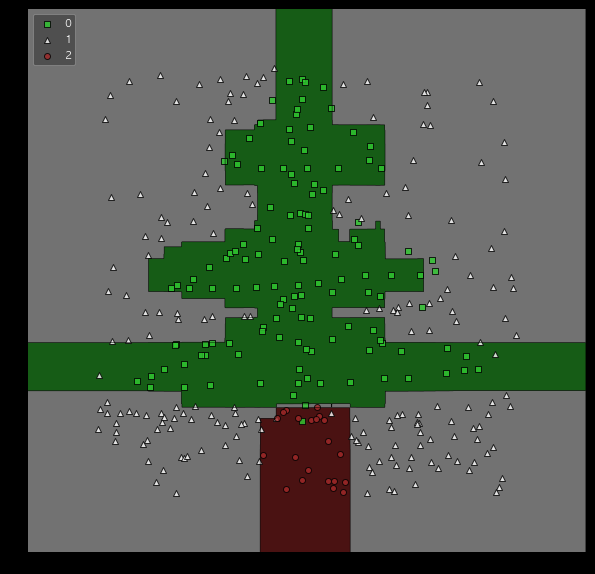u++の備忘録

# Christmas Tree Drawn with LightGBM

It is Christmas, so I painted Christmas tree with LightGBM.

This post is highly inspired by the following post:

The data was downloaded from the author's Github. And I added new data containing a new label representing the root of a tree.

```import random
random.seed(100)
x_add = [random.random() *6 - 3 for i in range(100)]
y_add = [-1 * random.random() *1.5 - 2.7 for i in range(100)]
label_add = [2 if abs(i) < 0.6 else 1 for i in x_add]

})

```

The plot_decision_regions of mlxtend library was used to draw decision boundaries of LightGBM. This is very easy to use, just passing the learned model and data.

```from mlxtend.plotting import plot_decision_regions
import lightgbm as lgb
from sklearn.model_selection import train_test_split

X = df[['x', 'y']]
y = df['label']
X_train, X_valid, y_train, y_valid= train_test_split(X, y, random_state = 0)
lgb_train = lgb.Dataset(X_train, y_train)
lgb_eval = lgb.Dataset(X_valid, y_valid, reference=lgb_train)

lgbm_params = {
'learning_rate': 0.2,
'num_leaves': 8,
'boosting_type': 'gbdt',
'reg_alpha': 1,
'reg_lambda': 1,
'objective': 'regression',
'metric': 'mae',
}

model = lgb.train(
lgbm_params, lgb_train,
valid_sets=lgb_eval,
num_boost_round=1000,
early_stopping_rounds=10,
)

plt.figure(figsize=(10,10))
plt.xticks(color="None")
plt.yticks(color="None")
plt.tick_params(length=0)
plot_decision_regions(np.array(X), np.array(y), clf=model, res=0.02, legend=2, colors='limegreen,white,brown')
```Merry Christmas!

The ipynb can be seen on my GitHub.